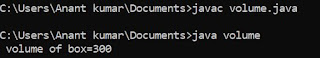## Sunday, 1 July 2018

### Array in C

An array is collection of homogenous element ( of same types ) consist a common property and share  a common name . An array is also known as collection of homogenous element from ( 0 to n-1 ) where n represent the maximum number of element of array .
An ordinary variable can contain only one value of one types it is not possible to assign more than one value at a single variables.

EXAMPLE :
Consider the following variable declaration and initialization .
int a = 10 ;
Here , a denote a single memory location with a value of 10.
If we update the value of variable .
a = 20 ;
Now the same memory location will contain the value 20 .

### Declaration of an array :

An array can be declared as an ordinary variable including the size specification in pair of [ ] bracket.

Syntax :   data type  variable name[ size ] ;
or
data type  variable name [n] ;
where n = number of elements

EXAMPLE
int a ;
In this example the name of an array is " a " which can contain maximum value of 3 at the 3 different locations .
These 3 different memory location are known as 3 element in c programming , these 3 element are also termed as the 3 subscript . that's why array is also known as " sub scripted variable" . The three different types of variable of a can be written as follows :
1 element : a 
2 element : a 
3 element : a 

### Initialization of an Array :

An array can be initialized in three different ways :
(1.)  Element by Element
Exam :   int a  ;
a = 22 ;
a = 5 ;
a = 66 ;

(2,) Declaration and initialization at same time
Exam :  int a  = { 11 , 22 , 66 } ;
it means
a  = 11 ;
a  = 22 ;
a  = 66 ;

(3.) Initialization from the user
Exam :
{
int i ;
int a  ;
for ( i = 0 ; i < 3 ; i++ )
{
printf ("\n Enter a number:") ;
scanf ("%d", & a [i]) ;
}

Output :
for ( i = 0 ; i < 3 ; i++ )
printf ("\n %d", a [i]) ;

### Program to Access  an array in C :

/* uses of array */
# include <stdio.h>
void main ()
{
int a , i ;
clrscr () ;
for ( i = 0 ; i < 5 ; i++ )
{
printf ("\n Enter a number:") ;
scanf ("%d", & a [i]) ;
}
for ( i = 0 ; i < 5 ; i++ )
printf ("\n %d", a[i]) ;
getch ()
}

### When above result is compiled :

Here as from loop statement is compiled then a condition of 5 is check out and a element of a to a is initialized from the user

#### 1 comment:

1.## Featured post

### Program to find Volume of given box in java

class volume { public static void main (String args []) { int l=5,b=6,h=10; int volume; volume=l*b*h; System.out.println (" vol...